Latest Banking jobs   »   Reasoning Ability Quiz For IBPS Clerk...

# Reasoning Ability Quiz For IBPS Clerk Prelims 2021- 26th July

Directions (1-3): Study the following information carefully and answer the question given below-
Seven members of a family are living in a house. There are three married couples and three generations in this family. D is grandmother of C, who is niece of L. A has only two children. B is sister-in-law of K. F is mother of C, who has no siblings. F is not sister of L.

Q1. If E is sister of B, then how L is related to E?
(a) Sister
(b) Brother
(c) Brother-in-law
(d) Father
(e) None of these

Q2. How A is related to F?
(a) Father
(b) Father-in-law
(c) Son
(d) Brother-in-law
(e) None of these

Q3. If M is son of L, then how M is related to D?
(a) Grandfather
(b) Nephew
(c) Grandson
(d) Son
(e) None of these

Q4. S is the only sister of O. H is the mother of S. K is married to O. H has only two sons. M is the niece of S and N. K has no sibling. S is unmarried. Then how is N related to K?
(a) Sister
(b) Son
(c) Brother
(d) Brother-in-law
(e) None of these

Q5. T’s mother is the sister-in-law of O and O is the only daughter of V. R is T’s brother. How is O related to R?
(a) Grandmother
(b) Aunt
(c) Sister
(d) Cousin
(e) Mother

Q6. Introducing a girl, a boy says, “She is the daughter of my father’s only brother-in-law”. How is the girl related to the boy?
(a) Sister-in-law
(b) Grand-daughter
(c) Cousin
(d) Daughter
(e) Aunt

Directions (7-10): Study the following information carefully and answer the question given below-
There are eight members in a family with three married couples. J is son-in-law of L, who is married to K. N has one son and one daughter. M is aunt of S. K is mother-in-law of G, who has no children. R is sister of S.

Q7. How J is related to R?
(a) Brother
(b) Uncle
(c) Grandfather
(d) Father
(e) None of these

Q8. Who among the following is sister-in-law of G?
(a) R
(b) N
(c) M
(d) J
(e) None of these

Q9. Which of the following statement is not true?
(a) R is daughter of N
(b) N is brother-in-law of G
(c) J is brother-in-law of M
(d) S is grandson of K
(e) Both (b) and (d)

Q10. How M is related to K?
(a) Daughter-in-law
(b) Niece
(c) Daughter
(d) Sister-in-law
(e) None of these

Directions (11-13): Each of these questions is based on the following information:
(i) A # B means A is the father of B.
(ii) A @ B means A is the mother of B.
(iii) A & B means A is the daughter of B.
(iv) A \$ B means A is the brother of B.
(v) A % B means A is the wife of B.

Q11. If the expression “P@U\$W%R#T\$Q” is true, then who among the following is uncle of Q?
(a) P
(b) U
(c) W
(d) R
(e) None of these

Q12. If the expression “E&G%H\$F#K&D” is true, then which of the following is not true?
(a) E is cousin of K
(b) F is aunt of E
(c) K is niece of H
(d) D is sister-in-law of H
(e) All are true

Q13. If the expression “M\$O&L%C#K@N” is true, then who among the following is grandmother of N?
(a) L
(b) O
(c) C
(d) M
(e) None of these

Directions (14-15): Study the following information carefully and answer the question given below-
There are eight persons in the family with two married couple. A is father of C. B is sister of F. E is mother of D. F is uncle of H. G is grandson of A. D is sister-in-law of B. C is father of H, who is granddaughter of E. D is unmarried. B has two children.

Q14. Who among the following brother-in-law of C?
(a) A
(b) E
(c) F
(d) G
(e) None of these

Q15. Who among the following daughter-in-law of A?
(a) H
(b) F
(c) G
(d) B
(e) None of these

Solutions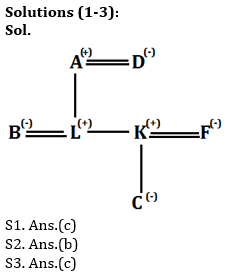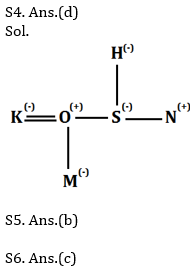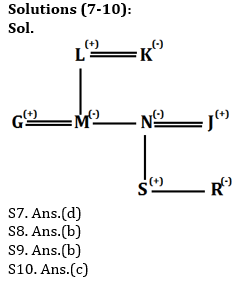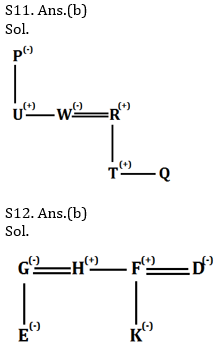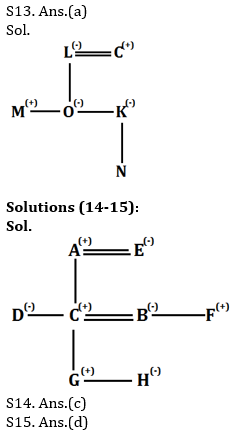#### Congratulations!Incorrect details? Fill the form again here

•Reasoning Ability Quiz For IBPS RRB PO C...
•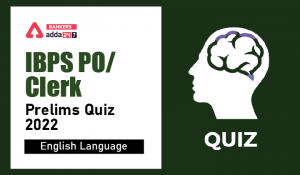English Quizzes For IBPS Clerk/PO Prelim...
•English Quizzes For IBPS Clerk/PO Prelim...
•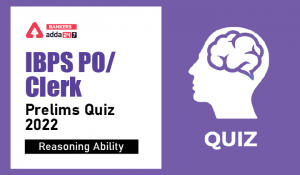Reasoning Ability Quiz For IBPS Clerk/PO...
•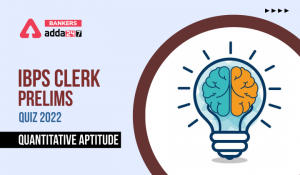Quantitative Aptitude Quiz For IBPS Cler...
•Quantitative Aptitude Quiz For IBPS Cler...